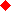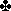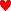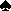Main   Exercise 3U53 by Richard PavlicekChoose the best description of each queried rebid by East from:
S = Sign-off, N = Nonforcing, I = Invitational, F = Forcing
A = Artificial force, G = Gerber, B = Blackwood

 1.West1 NT2NorthPassPass East2? SouthPass

What is 2?

What is 3?

What is 3?

What is 4?

What is 4?

What is 4 NT?

 2.West12NorthPassPass East1? SouthPass

What is 2?

What is 2?

What is 2 NT?

What is 3?

What is 3?

What is 4 NT?TopMain

 3.West11NorthPassPass East1? SouthPass

What is 2?

What is 2?

What is 2?

What is 2 NT?

What is 3?

What is 3?

 4.West11 NT NorthPassPass East1? SouthPass

What is 2?

What is 2?

What is 2?

What is 2 NT?

What is 3?

What is 4?TopMain

 5.West13NorthPassPass East1? SouthPass

What is 3?

What is 3?

What is 3 NT?

What is 4?

What is 4 NT?

What is 5?

 6.West12NorthPassPass East2? SouthPass

What is 2?

What is 2 NT?

What is 3?

What is 3?

What is 3?

What is 4?TopMain